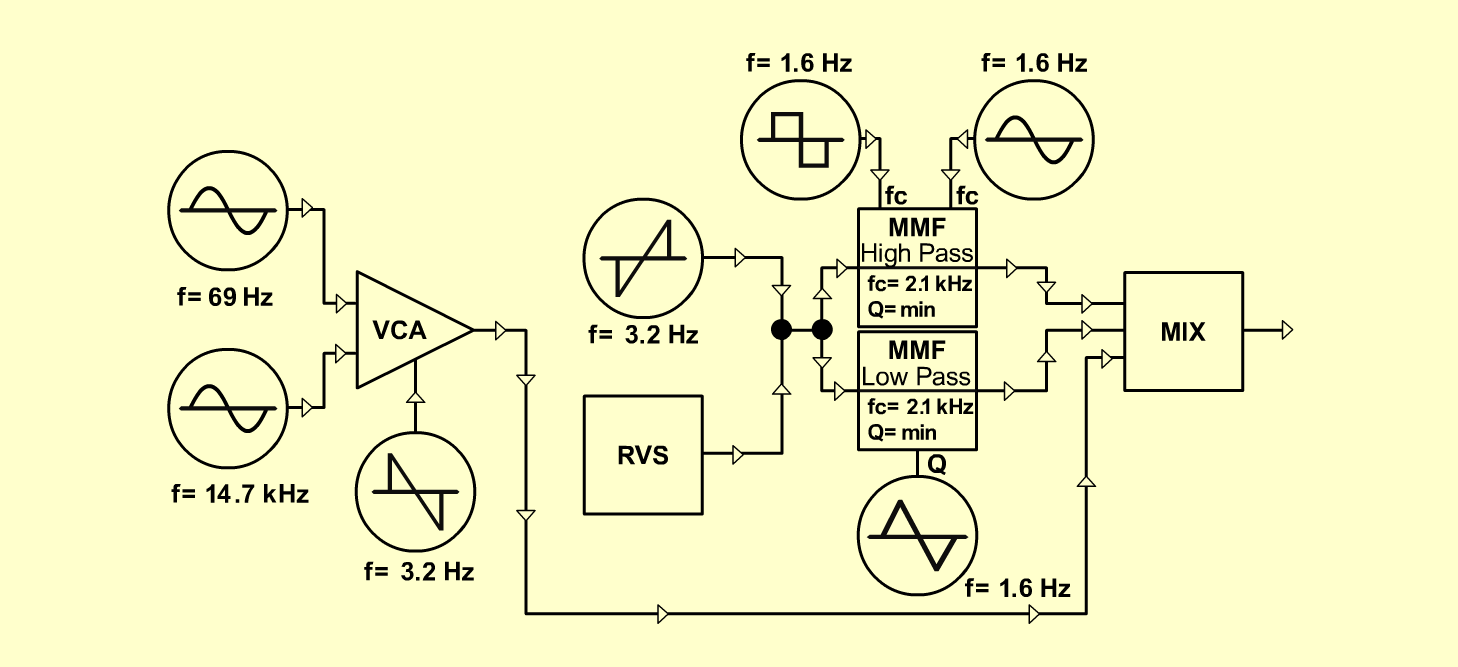D o e p f e r A - 100 Connections: Settings: A-110/1 (Sine)  <=>  A-131 (Audio In 1) A-110/2 (Sine)  <=>  A-131 (Audio In 2) A-176 (CV 3)  <=>  A-110/2 (CV 1) A-145 (Saw down)  <=>  A-131 (CV 1) A-145 (Saw up)  <=>  A-180 A-145 (Sine)  <=>  A-121 (FCV 2) A-145 (Triangle)  <=>  A-121 (QCV 2) A-145 (Square)  <=>  A-121 (FCV 1) A-118 (Random)  <=>  A-180 A-180  <=>  A-121 (Audio In) A-121 (High)  <=>  A-138 (Input 1) A-121 (Low)  <=>  A-138 (Input 2) A-131 (Audio Out)  <=>  A-138 (Input 3) A-138 (Output)  <=> Amplifier A-110/1 (Range = 0, Tune = 10) A-110/2 (Range = +2, Tune = 10) A-118 (Blue = 10, Red = 0, Rate = 0, Level = 3) A-176 (CV 3 = 10) A-121 (Audio Level = 10, Freq = 6, FCV 2 = 10, Res = 0, QCV 2 = 10) A-131 (Gain = 10, Audio In 1 = 0, Audio In 2 = 0,  Audio Out = 0) A-138 (In 1 = 10, In 2 = 0, In 3 = 0, Out = 10) A-145 (Frq = 8, Range = L) Notes: Set the following knobs manually: A-118 (Level), A-121 (Freq, QCV2), A-131 (In 1, In 2, In 3), A-138 (In 2, In 3) Markus Decker, September 2001

 Patch \ Modul A-118 A-121 A-138 A-138 A-131 A-131 A-131 Level Freq Input 2 Input 3 In 1 In 2 In 3 Audioroom 1 3 6 0 0 0 0 0 Audioroom 2 10 6 5 5 0 10 10 Audioroom 3 10 6 10 3 0 10 10 Audioroom 4 10 7 10 4 5 10 10Sound samples Audioroom 1 Audioroom 2 Audioroom 3 Audioroom 4